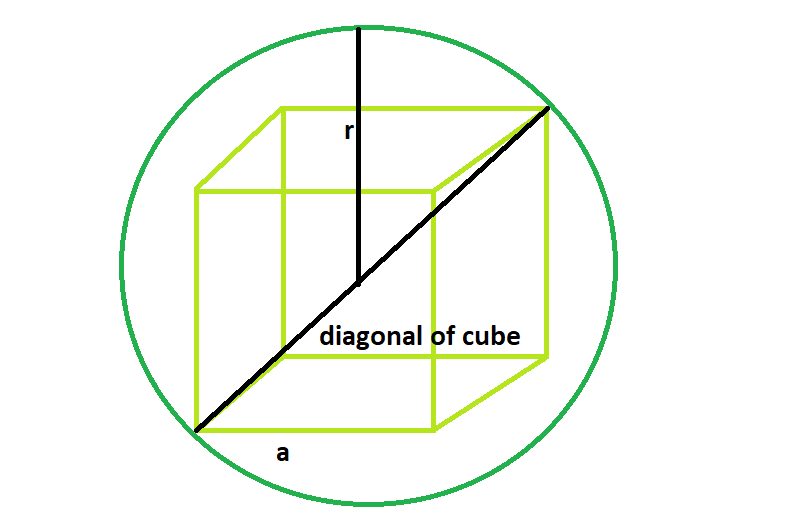GeeksforGeeks App
Open AppBrowser
Continue

# Largest cube that can be inscribed within the sphere

Given here is a sphere of radius r, the task is to find the side of the largest cube that can fit inside in it.
Examples:

```Input: r = 8
Output: 9.2376

Input: r = 5
Output: 5.7735```Approach:

Side of the cube = a
Radius of the sphere = r
From the diagonal, it is clear that, diagonal of the cube = diameter of the sphere,
a√3 = 2r or, a = 2r/√3

Below is the implementation:

## C++

 `// C++ Program to find the biggest cube``// inscribed within a sphere``#include ``using` `namespace` `std;` `// Function to find the side of the cube``float` `largestCube(``float` `r)``{` `    ``// radius cannot be negative``    ``if` `(r < 0)``        ``return` `-1;` `    ``// side of the cube``    ``float` `a = (2 * r) / ``sqrt``(3);``    ``return` `a;``}` `// Driver code``int` `main()``{``    ``float` `r = 5;``    ``cout << largestCube(r) << endl;` `    ``return` `0;``}`

## Java

 `// Java Program to find the biggest cube``// inscribed within a sphere``import` `java.util.*;``class` `Solution{``// Function to find the side of the cube``static` `float` `largestCube(``float` `r)``{``  ` `    ``// radius cannot be negative``    ``if` `(r < ``0``)``        ``return` `-``1``;``  ` `    ``// side of the cube``    ``float` `a = (``2` `* r) / (``float``)Math.sqrt(``3``);``    ``return` `a;``}``  ` `// Driver code``public` `static` `void` `main(String args[])``{``    ``float` `r = ``5``;``    ``System.out.println( largestCube(r));``  ` `}` `}``//contributed by Arnab Kundu`

## Python3

 `# Python 3 Program to find the biggest``# cube inscribed within a sphere``from` `math ``import` `sqrt` `# Function to find the side of the cube``def` `largestCube(r):``    ` `    ``# radius cannot be negative``    ``if` `(r < ``0``):``        ``return` `-``1` `    ``# side of the cube``    ``a ``=` `(``2` `*` `r) ``/` `sqrt(``3``)``    ``return` `a` `# Driver code``if` `__name__ ``=``=` `'__main__'``:``    ``r ``=` `5``    ``print``(``"{0:.6}"``.``format``(largestCube(r)))` `# This code is contributed``# by SURENDRA_GANGWAR`

## C#

 `// C# Program to find the biggest cube``// inscribed within a sphere``using` `System;``class` `Solution{``// Function to find the side of the cube``static` `float` `largestCube(``float` `r)``{` `    ``// radius cannot be negative``    ``if` `(r < 0)``        ``return` `-1;` `    ``// side of the cube``    ``float` `a = (2 * r) / (``float``)Math.Sqrt(3);``    ``return` `a;``}` `// Driver code``static` `void` `Main()``{``    ``float` `r = 5;``    ``Console.WriteLine( largestCube(r));` `}` `}``//This code is contributed by mits`

## PHP

 ``

## Javascript

 ``

Output:

`5.7735`

Time Complexity: O(1)

Auxiliary Space: O(1)

My Personal Notes arrow_drop_up